## Sunday, April 24, 2011

### Weekly 3LB Update for week ending 4/22/11

Weekly 3LB update (week ending 4/22/11)
a new high or low will change the reversal price and the midpoint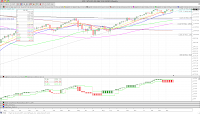SPX
-no change (above mid)
trend=no
direction=down (1 bar)
low= 1279.20
rev= 1343.01; mid= 1311.11

monthly info -no change (above mid)
trend=up
high= 1327.22
rev= 1089.41; mid= 1208.32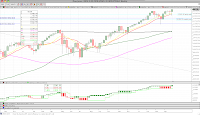Dow Jones
WEEKLY REVERSAL new high 12505.99
trend=no
direction=up (1 bar)
high= 12505.99
rev= 11858.52; mid= 12182.26

monthly info new high 12319.73
trend=up
high= 12319.73
rev= 11577.51; mid= 11948.62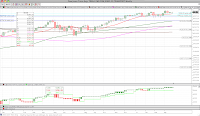Dow Jones Transportation Avg
-no change (above mid)
trend=up
high= 5370.47
rev= 5045.62; mid= 5208.05

monthly info new high 5299.89
trend=up
high= 5299.89
rev= 4754.29; mid= 5027.09Nasdaq Composite
-no change (above mid)
trend=no
direction=down (2 bars)
low= 2643.67
rev= 2833.95; mid= 2738.81

monthly info -no change (above mid)
trend=up
high= 2782.27
rev= 2507.41; mid= 2644.84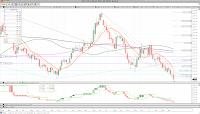DXY
new low 74.11
trend=down
low= 74.11
rev= 75.72; mid= 74.92

monthly info new low 75.86
trend=down
low= 75.86
rev= 78.72; mid= 77.29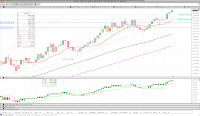Gold
new high 1503.80
trend=up
high= 1503.80
rev= 1429.90; mid= 1466.85

monthly info new high 1439.90
trend=up
high= 1439.90
rev= 1362.20; mid= 1401.05VIX
new low 14.69
trend=down
low= 14.69
rev= 16.11; mid= 15.40

monthly info -no change
trend=no; direction=up (2 bars)
high= 34.54
rev= 17.59; mid= 26.07WTI Crude Oil
-no change (above mid)
trend=up
high= 113.38
rev= 105.98; mid= 109.68

monthly info MONTHLY REVERSAL new high 106.72
trend=down
high= 106.72
rev= 79.74; mid= 93.23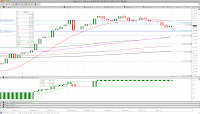Sugar #11
-no change (below mid)
trend=no
direction=up (2 bars)
high= 30.92
rev= 23.09; mid= 27.01

monthly info -no change (below mid)
trend=up
high= 33.97
rev= 22.73; mid= 28.35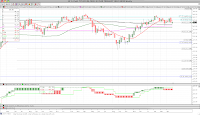30 Year Yield
-no change (below mid)
trend=no
direction=down (1 bar)
low= 44.29
rev= 47.37; mid= 45.83

monthly info -no change
trend=up; direction=up (2 bars)
high= 47.15
rev= 26.91; mid= 37.03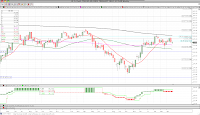10 Year Yield
-no change (below mid)
trend=no
direction=down (1 bar)
low= 32.77
rev= 36.52; mid= 34.65

monthly info -no change
trend=no; direction=up (1 bar)
high= 38.43
rev= 22.44; mid= 30.44Silver
new high 46.06
trend=up
high= 46.06
rev= 37.74; mid= 41.90

monthly info new high 37.89
trend=up
high= 37.89
rev= 28.21; mid= 33.05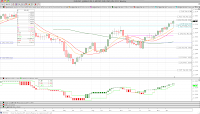EURUSD
new high 1.4555
trend=up
high= 1.4555
rev= 1.4216; mid= 1.4386

monthly info new high 1.4181
trend=up
high= 1.4181
rev= 1.2302; mid= 1.3242GS (Goldman Sachs)
WEEKLY CONFIRMATION new low 153.51
trend=no
direction=down (2 bars)
low= 153.51
rev= 175.00; mid= 164.26

monthly info -no change - bearish thrusting
trend=no; direction=up (2 bars)
high= 184.35
rev= 78.99; mid= 131.67LUMBER
new low 231.00
trend=down
low= 231.00
rev= 337.10; mid= 284.05

monthly info -no change - bearish thrusting
trend=no; direction=up (1 bar)
high= 316.10
rev= 223.00; mid= 269.55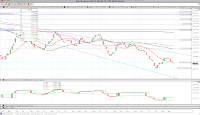BALTIC DRY INDEX
-no change (below mid)
trend=no
direction=up (2 bars)
high= 1585.00
rev= 1043.00; mid= 1314.00

monthly info - no change
trend=down
low= 715.00
rev= 9370.00; mid= 5042.50XLF (SPDR Financial)
WEEKLY CONFIRMATION new low 16.10
trend=no
direction=down (2 bars)
low= 16.10
rev= 17.18; mid= 16.64

monthly info MONTHLY CONFIRMATION new high 16.85
trend=no; direction=up (2 bars)
high= 16.85
rev= 13.55; mid= 15.20AUDJPY
-no change (above mid)
trend=up
high= 0.8864
rev= 0.8241; mid= 0.8553

monthly info MONTHLY REVERSAL new high 0.8522
trend=up
high= 0.8522
rev= 0.7114; mid= 0.7818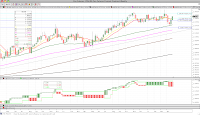YEN
-no change (above mid)
trend=no
direction=down (1 bar)
low= 1.17910
rev= 1.24100; mid= 1.21005

monthly info -no change
trend=up
high= 1.24330
rev= 1.15910; mid= 1.20120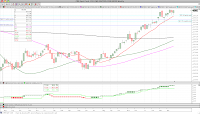CRB
-no change (above mid)
trend=up
high= 368.70
rev= 341.78; mid= 355.24

monthly info new high 359.43
trend=up
high= 359.43
rev= 332.80; mid= 346.12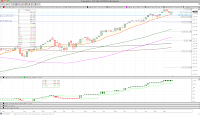CAT (Caterpillar)
-no change (above mid)
trend=up
high= 113.12
rev= 103.54; mid= 108.33

monthly info new high 111.35
trend=up
high= 111.35
rev= 93.66; mid= 102.51

BIDU (Baidu)
new high 148.65
trend=up
high= 148.65
rev= 138.83; mid= 143.74

JPM (JP Morgan Chase)
WEEKLY CONFIRMATION new low 44.68
trend=no
direction=down (2 bars)
low= 44.68
rev= 48.00; mid= 46.34

BAC (Bank of America)
WEEKLY REVERSAL new low 12.31
trend=no
direction=down (2 bars)
low= 12.31
rev= 15.25; mid= 13.78

AXP (American Express)
-no change (above mid)
trend=no
direction=down (2 bars)
low= 37.99
rev= 48.05; mid= 43.02

AMZN (Amazon)
-no change (above mid)
trend=no
direction=down (2 bars)
low= 161.82
rev= 189.25; mid= 175.54

M (Macy's)
-no change (below mid)
trend=down
low= 22.82
rev= 25.57; mid= 24.20

BKX (KBW Bank Index)
new low 50.47
trend=down
low= 50.47
rev= 52.09; mid= 51.28

Powershares USD Bull (UUP)
new low 21.24
trend=down
low= 21.24
rev= 21.72; mid= 21.48

TLT (iShares Barclays 20+ Year Treas Bond)
-no change (above mid)
trend=no
direction=up (1 bar)
high= 93.46
rev= 88.81; mid= 91.14

AAPL (Apple Computer)
-no change (above mid)
trend=no
direction=down (2 bars)
low= 327.46
rev= 360.00; mid= 343.73

V (Visa)
-no change (above mid)
trend=no
direction=down (1 bar)
low= 66.90
rev= 80.20; mid= 73.55

XRT (SPDR Retail ETF)
new high 52.80
trend=up
high= 52.80
rev= 51.06; mid= 51.93

MUB (iShares S&P National AMT-Fre)
-no change (above mid)
trend=down
low= 96.26
rev= 101.61; mid= 98.94

IQI (Morgan Stanley Quality Muni)
-no change (above mid)
trend=down
low= 11.19
rev= 12.32; mid= 11.76

JNK (SPDR Barclays Capital HY)
-no change (above mid)
trend=no
direction=down (1 bar)
low= 39.70
rev= 41.12; mid= 40.41

COPPER
-no change (above mid)
trend=up
high= 4.580
rev= 4.159; mid= 4.370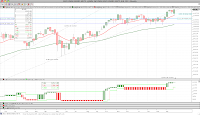MSCI Emerging Markets
new high 1212.20
trend=up
high= 1212.20
rev= 1122.20; mid= 1167.20

monthly info new high 1168.10
trend=up
high= 1168.10
rev= 1074.20; mid= 1121.15NIKKEI
-no change (below mid)
trend=down
low= 9206.75
rev= 10605.65; mid= 9906.20

monthly info -no change
trend=no; direction=down (1 bar)
low= 8824.06
rev= 11089.94; mid= 9957.00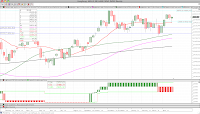HANG SENG
-no change (above mid)
trend=no
direction=down (1 bar)
low= 22300.23
rev= 24876.82; mid= 23588.53

monthly info new high 23527.52
trend=up
high= 23527.52
rev= 22358.17; mid= 22942.85SHANGHAI Composite
-no change (above mid)
trend=up
high= 3050.52
rev= 2942.31; mid= 2996.42

monthly info -no change
trend=no; direction=up (2 bars)
high= 3412.06
rev= 1728.79; mid= 2570.43KOSPI
new high 2198.54
trend=up
high= 2198.54
rev= 2121.01; mid= 2159.78

monthly info new high 2106.70
trend=up
high= 2106.70
rev= 1904.63; mid= 2005.67Athens
new low 1431.33
trend=down
low= 1431.33
rev= 1661.68; mid= 1546.51

monthly info -no change
trend=no; direction=up (1 bar)
high= 1593.30
rev= 1413.94; mid= 1503.62